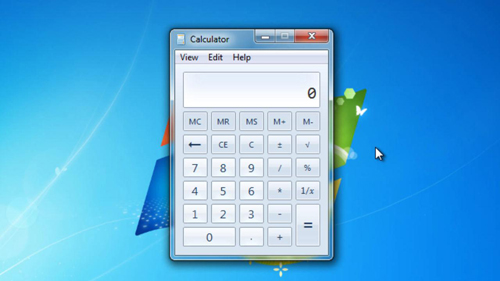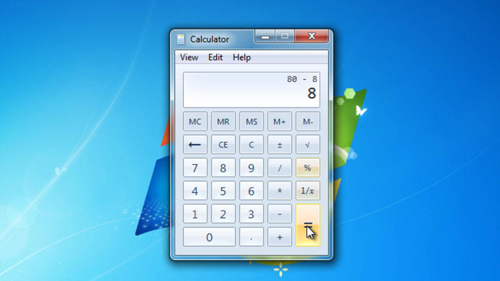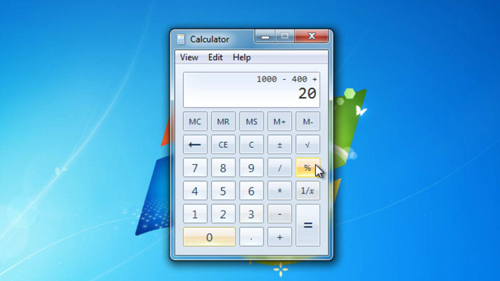## How to Calculate Percentage on Computer’s Calculator

This tutorial will teach you how to calculate percentage in Windows calculator.

Step # 1 – Percentage Increases
First you need to open the calculator program, make sure it is in the standard (not scientific) view. As you can see here it is. The first calculation we are going to look at is how to add a percentage amount to a figure, for example 100 +10% of that figure. To do this you need to enter “100” then click the add key and then enter “10” and click the percent key. This will convert the sum to “100+10” which is clearly correct, just click the equals sign to complete the sum.Step # 2 – Percentage Decreases
Now that you know how the percentage key works for an increase it is fairly logical how a decrease works. For example let’s say we want to reduce 80 by 10%, enter “80” then click minus then click “10” and then the percentage key. Finally click equals and the result will appear, here, “72”.Step # 3 – Combination of Percentages
Let’s have a look at how we can combine these percentage calculations with a real world example. Let’s say we have a bicycle for sale for \$1000, it is on sale by 40%, but it also attracts a sales tax of 20%. Working out the final price with calculator is very easy. Enter the original price, here 1000, then minus “40” with the percent button, then you need to add the tax so click the plus key then type 20 and then the percentage sign. Finally click the equals key and you will be given your final cost, here that is \$720 – which is correct. You now know how to calculate percentage using Windows calculator.